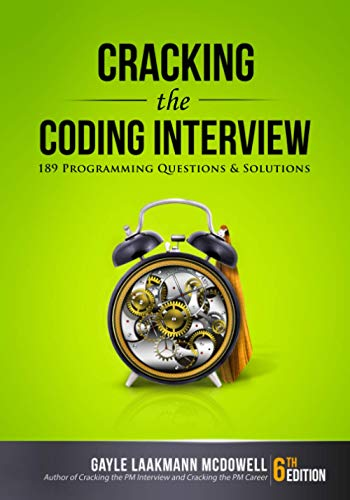# Bulb Or No Bulb?

Question: There are 100 bulbs arranged in a row. Each bulb has its own switch and is currently turned off.  In the first round, you turn every switch on. In the second round, you flip the switch of every second bulb (i.e. bulb 2, 4, 6, 8 and so on). In the third round, you flip the switch of every third bulb and so on. What is the state of bulb 9 after 100 rounds? Also, how many bulbs are on after 100 rounds?

Answer: Sounds easy enough? Let’s take our usual methodical approach.

Lets think about the round in which each bulb gets flipped. In round 2, every even numbered bulb gets flipped. In round 10, every bulb that ends in a zero will get flipped.

So lets pick bulb 48 per say. It gets flipped in rounds 1, 2, 3, 4, 6, 8, 12, 16, 24 and 48. So essentially every round thats a factor of 48. If you notice carefully, you will see that these factors always come in pairs like (1, 48), (2, 24), (3, 16) and so on. Since they occur in pairs, for each flip that turns the bulb on, there is a flip that turns it off.

But what happens to bulb 81. So if we find the factors of 81, we get (1, 81), (3, 27) and (9, 9). But round 9 only happens once. So for every number that has a pair of factors that are equal (a.k.a perfect squares), there will be an odd number of flips. So this leaves all bulbs off except those bulbs which are perfect squares.

Since 9 is a perfect square, it will be ON after 100 rounds. Also, since there are 10 perfect squares from 1 to 100 (bulbs 1, 4, 9, 16, 25, 36, 49, 64, 81, 100), there will be 10 bulbs that remain ON after round 100.

If you're looking for some serious preparation for your interviews, I'd recommend this book written by a lead Google interviewer. It has 189 programming questions and solutions:## One Response

1.Mohaideen at SKCET says:

the above solution implies , tat the no of bulb left after the entire process is equal to no of perfect square wit in 100

XHTML: These are some of the tags you can use: `<a href=""> <b> <blockquote> <code> <em> <i> <strike> <strong>`